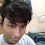# Random Gradient Generator With CSS and Javascript

Let's see how to generate random gradient background using JavaScript. This is very simple. We will use Math.random() of JavaScript to generate any random value we want to generate. We will have some functions in JavaScript :

1) randomElement() : To generate a random value between 0 to f or the hexadecimal digits.

2) randomColor() : To generate a random Hex color.

3) randomAngle() : To generate a random angle between 0 to 360.

4) applyBg() : To apply the background gradient to a particular element.

Now , Let's see the live preview of our project.

Let's understand in short how is it generating random gradient everytime. We have an inbuilt function in JavaScript called Math.rand() which generates random fraction value between 0 to 1. So if I multiply that with any number N and floor or round off the value, it will output any random value between 0 to N in integer format. This concept we have used here.

To generate the color code at the very beginning we created an array of all hex digits. And then randomly selected one of them with random index in randomElement function. Then repeated this this six times and created a color code in randomColor function. randomAngle is simply a random integer generation process between 0 to 360, it's working process will be similar as index generation. Then we will generate multiple random color and create the gradient in random angle and finally apply it to a particular element's background. This is as simple as I explained.   I will give you the complete code here, You can copy the code from here :

``````<!DOCTYPE html>
<html lang="en">
<meta charset="UTF-8" />
<meta http-equiv="X-UA-Compatible" content="IE=edge" />
<meta name="viewport" content="width=device-width, initial-scale=1.0" />
<style>
* {
margin: 0;
box-sizing: border-box;
}

body .my-container {
height: 100vh;
width: 100%;
background: #ff9;
position: relative;
}

button#btn {
position: absolute;
left: 50%;
bottom: 60px;
transform: translateX(-50%);
font-size: 18px;
color: #fff;
background: #000;
border: none;
outline: none;
cursor: pointer;

}

.code-element-item {
position: absolute;
left: 50%;
bottom: 30px;
transform: translateX(-50%);
font-size: 18px;
color: #000;
background-color: #fff;
font-family: consolas, sans-serif;
}
</style>
<title>Document</title>
<body>
<div class="my-container">
<button id="btn">Change Background</button>
<span class="code-element-item"></span>
</div>
<script>
const container = document.querySelector(".my-container");
const codeEl = document.querySelector(".code-element-item");

const btn = document.getElementById("btn");

var array = [0, 1, 2, 3, 4, 5, 6, 7, 8, 9, "a", "b", "c", "d", "e", "f"];

function randomElement() {
return array[Math.floor(array.length * Math.random())];
}

function randomColor() {
let colour = "#";
for (var i = 0; i < 6; i++) {
colour += randomElement();
}

return colour;
}

function randomAngle() {
return Math.floor(360 * Math.random());
}

btn.onclick = applyBg;

applyBg();

function applyBg() {
let newBg =
randomAngle() +
"deg, " +
randomColor() +
", " +
randomColor() +
", " +
randomColor() +
")";
container.style.background = newBg;
codeEl.textContent = newBg;
}
</script>
</body>
</html>

``````

Hope, you have understood this post and make sure to comment your opinions and suggestions and problems. Mention your country also.

You may also like to read - Random Jokes Generator Using JavaScript and a free API

Thank you . The end..### Imon Raj

Software, Web & Android Developer

##### My Skills:

1.Love from INDIA ,❤️❤️

2.Great content 👍 I really enjoyed with your knowledge and development ideas.

1.Thank you very much. Keep supporting :)